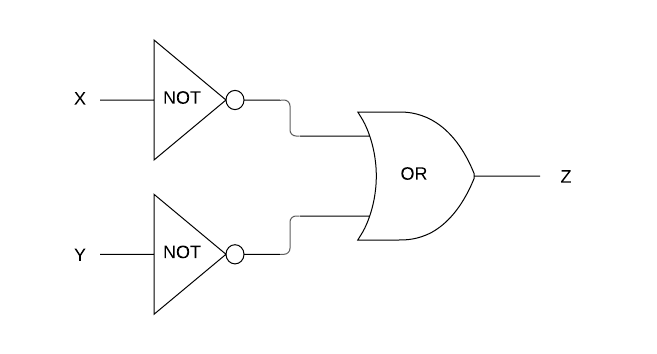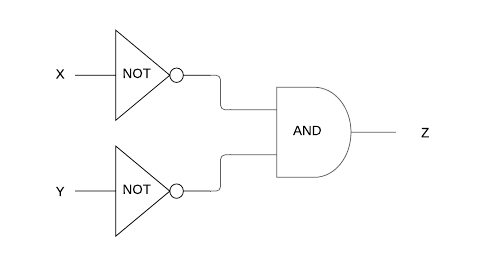Question 1[6 Marks]
Consider the following boolean expression:
(A AND NOT B) OR (B AND NOT A)
(a).
Construct a truth table for the boolean expression above.

(b).
Hence or otherwise, state the simple boolean expression composed of only one logical operator which is logically equivalent to the boolean expression above.

Question 2[4 Marks]
(a).
Construct a truth table for the logic diagram represented below.(b).
What single gate is logically equivalent to the configuration represented in part (a)?

(c).
If, in part (a), the OR gate was replaced by an AND gate to produce the logic diagram below, what single gate would it then become logically equivalent to?Question 3[4 Marks]
Construct both a logic diagram and truth table for the following expression:
NOT A OR A AND B
Question 4[4 Marks]
Construct both a logic diagram and truth table for the following expression:
A AND (A OR NOT B)

Login or Create an Account to view question mark schemes, comment, and download a PDF of this test
HomeThe Right Test - Fast